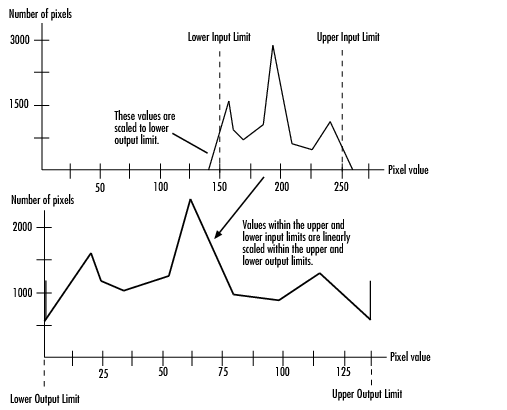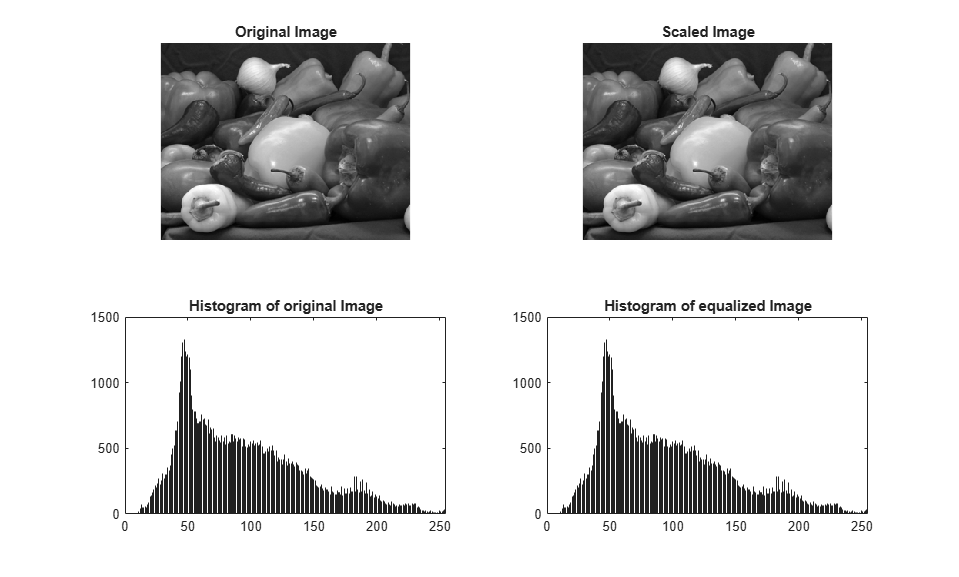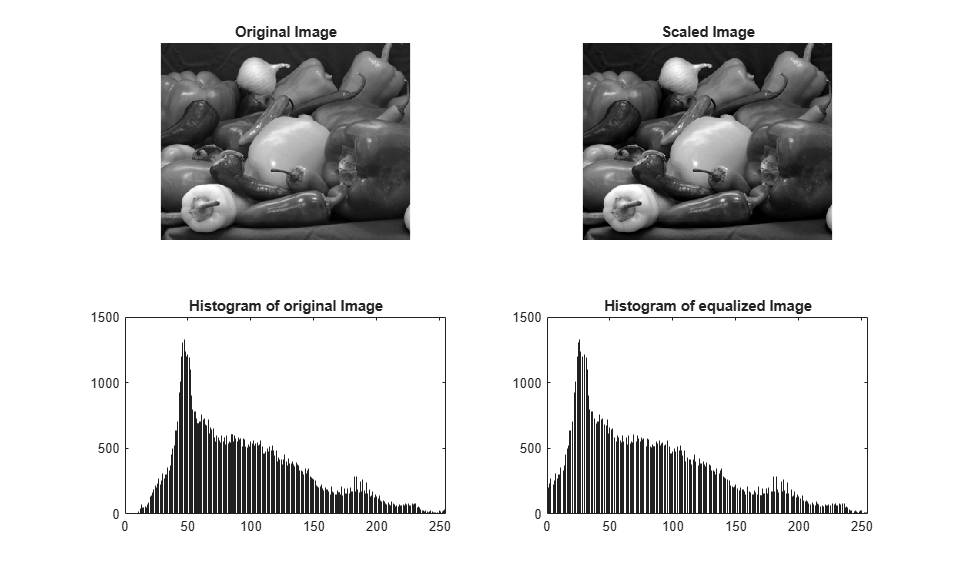# SystemC Code Generation for Contrast Adjustment

This example shows how to generate SystemC code from a MATLAB® design that adjusts image contrast by linearly scaling pixel values.

### Algorithm

Contrast adjustment adjusts the contrast of an image by linearly scaling the pixel values between upper and lower limits. Pixel values that are above or below this range are saturated to the upper or lower limit value, respectively.### MATLAB Design

```design_name = 'mlhdlc_image_scale'; testbench_name = 'mlhdlc_image_scale_tb'; ```

Review the MATLAB design:

```type(design_name); ```
```%%%%%%%%%%%%%%%%%%%%%%%%%%%%%%%%%%%%%%%%%%%%%%%%%%%%%%%%%%%%%%%%%%%%%%%%%%% % scale.m % % Adjust image contrast by linearly scaling pixel values. % % The input pixel value range has 14bits and output pixel value range is % 8bits. % %%%%%%%%%%%%%%%%%%%%%%%%%%%%%%%%%%%%%%%%%%%%%%%%%%%%%%%%%%%%%%%%%%%%%%%%%%% function [x_out, y_out, pixel_out] = ... mlhdlc_image_scale(x_in, y_in, pixel_in, ... damping_factor_in, dynamic_range_in, ... tail_size_in, max_gain_in, ... width, height) % Copyright 2011-2022 The MathWorks, Inc. persistent histogram1 histogram2 persistent low_count persistent high_count persistent offset persistent gain persistent limits_done persistent damping_done persistent reset_hist_done persistent scaling_done persistent hist_ind persistent tail_high persistent min_hist_damped %Damped lower limit of populated histogram persistent max_hist_damped %Damped upper limit of populated histogram persistent found_high persistent found_low DR_PER_BIN = 8; SF = 1./(1:(2^14/8)); % be nice to fix this NR_OF_BINS = (2^14/DR_PER_BIN) - 1; MAX_DF = 255; if isempty(offset) offset = 1; gain = 1; limits_done = 1; damping_done = 1; reset_hist_done = 1; scaling_done = 1; hist_ind = 1; tail_high = NR_OF_BINS; low_count = 0; high_count = 0; min_hist_damped = 0; max_hist_damped = (2^14/DR_PER_BIN) - 1; found_high = 0; found_low = 0; end if isempty(histogram1) histogram1 = zeros(1, NR_OF_BINS+1); histogram2 = zeros(1, NR_OF_BINS+1); end if y_in < height frame_valid = 1; if x_in < width line_valid = 1; else line_valid = 0; end else frame_valid = 0; line_valid = 0; end % initialize at beginning of frame if x_in == 0 && y_in == 0 limits_done = 0; damping_done = 0; reset_hist_done = 0; scaling_done = 0; low_count = 0; high_count = 0; hist_ind = 1; end max_gain_frac = max_gain_in/2^4; pix11 = floor(pixel_in/DR_PER_BIN); pix_out_temp = pixel_in; %************************************************************************** %Check if valid part of frame. If pixel is valid remap pixel to desired %output dynamic range (dynamic_range_in) by subtracting the damped offset %(min_hist_damped) and applying the calculated gain calculated from the %previous frame histogram statistics. %************************************************************************** % histogram read histReadIndex1 = 1; histReadIndex2 = 1; if frame_valid && line_valid histReadIndex1 = pix11+1; histReadIndex2 = pix11+1; elseif ~limits_done histReadIndex1 = hist_ind; histReadIndex2 = NR_OF_BINS - hist_ind; end histReadValue1 = histogram1(histReadIndex1); histReadValue2 = histogram2(histReadIndex2); histWriteIndex1 = NR_OF_BINS+1; histWriteIndex2 = NR_OF_BINS+1; histWriteValue1 = 0; histWriteValue2 = 0; if frame_valid if line_valid temp_sum = histReadValue1 + 1; ind = min(pix11+1, NR_OF_BINS); val = min(temp_sum, tail_size_in); histWriteIndex1 = ind; histWriteValue1 = val; histWriteIndex2 = ind; histWriteValue2 = val; %Scale pixel pix_out_offs_corr = pixel_in - min_hist_damped*DR_PER_BIN; pix_out_scaled = pix_out_offs_corr * gain; pix_out_clamp = max(min(dynamic_range_in, pix_out_scaled), 0); pix_out_temp = pix_out_clamp; end else %********************************************************************** %Ignore tail_size_in pixels and find lower and upper limits of the %histogram. %********************************************************************** if ~limits_done if hist_ind == 1 tail_high = NR_OF_BINS-1; offset = 1; found_high = 0; found_low = 0; end low_count = low_count + histReadValue1; hist_ind_high = NR_OF_BINS - hist_ind; high_count = high_count + histReadValue2; %Found enough high outliers if high_count > tail_size_in && ~found_high tail_high = hist_ind_high; found_high = 1; end %Found enough low outliers if low_count > tail_size_in && ~found_low offset = hist_ind; found_low = 1; end hist_ind = hist_ind + 1; %All bins checked so limits must already be found if hist_ind >= NR_OF_BINS hist_ind = 1; limits_done = 1; end %********************************************************************** %Damp the limit change to avoid image flickering. Code below equivalent %to: max_hist_damped = damping_factor_in*max_hist_dampedOld + %(1-damping_factor_in)*max_hist_dampedNew; %********************************************************************** elseif ~damping_done min_hist_weighted_old = damping_factor_in*min_hist_damped; min_hist_weighted_new = (MAX_DF-damping_factor_in+1)*offset; min_hist_weighted = (min_hist_weighted_old + ... min_hist_weighted_new)/256; min_hist_damped = max(0, min_hist_weighted); max_hist_weighted_old = damping_factor_in*max_hist_damped; max_hist_weighted_new = (MAX_DF-damping_factor_in+1)*tail_high; max_hist_weighted = (max_hist_weighted_old + ... max_hist_weighted_new)/256; max_hist_damped = min(NR_OF_BINS, max_hist_weighted); damping_done = 1; hist_ind = 1; %********************************************************************** %Reset all bins to zero. More than one bin can be reset per function %call if blanking time is too short. %********************************************************************** elseif ~reset_hist_done histWriteIndex1 = hist_ind; histWriteValue1 = 0; histWriteIndex2 = hist_ind; histWriteValue2 = 0; hist_ind = hist_ind+1; if hist_ind == NR_OF_BINS reset_hist_done = 1; end %********************************************************************** %The gain factor is determined by comparing the measured damped actual %dynamic range to the desired user specified dynamic range. Input %dynamic range is measured in bins over DR_PER_BIN space. %********************************************************************** elseif ~scaling_done dr_in = round(max_hist_damped - min_hist_damped); gain_temp = dynamic_range_in*SF(dr_in); gain_scaled = gain_temp/DR_PER_BIN; gain = min(max_gain_frac, gain_scaled); scaling_done = 1; hist_ind = 1; end end histogram1(histWriteIndex1) = histWriteValue1; histogram2(histWriteIndex2) = histWriteValue2; x_out = x_in; y_out = y_in; pixel_out = pix_out_temp; ```
```type(testbench_name); ```
```%Test bench for scaling, analogous to automatic gain control (AGC) % Copyright 2011-2022 The MathWorks, Inc. testFile = 'mlhdlc_img_peppers.png'; imgOrig = imread(testFile); [height, width] = size(imgOrig); imgOut = zeros(height,width); hBlank = 20; % make sure we have enough vertical blanking to filter the histogram vBlank = ceil(2^14/(width+hBlank)); %df - Temporal damping factor of rescaling %dr - Desired output dynamic range df = 0; dr = 255; nrOfOutliers = 248; maxGain = 2*2^4; for frame = 1:2 disp(['frame: ', num2str(frame)]); for y_in = 0:height+vBlank-1 %disp(['frame: ', num2str(frame), ' of 2, row: ', num2str(y_in)]); for x_in = 0:width+hBlank-1 if x_in < width && y_in < height pixel_in = double(imgOrig(y_in+1, x_in+1)); else pixel_in = 0; end [x_out, y_out, pixel_out] = ... mlhdlc_image_scale(x_in, y_in, pixel_in, df, dr, ... nrOfOutliers, maxGain, width, height); if x_out < width && y_out < height imgOut(y_out+1,x_out+1) = pixel_out; end end end figure('Name', [mfilename, '_scale_plot']); imgOut = round(255*imgOut/max(max(imgOut))); subplot(2,2,1); imshow(imgOrig, []); title('Original Image'); subplot(2,2,2); imshow(imgOut, []); title('Scaled Image'); subplot(2,2,3); histogram(double(imgOrig(:)),2^14-1); axis([0, 255, 0, 1500]); title('Histogram of original Image'); subplot(2,2,4); histogram(double(imgOut(:)),2^14-1); axis([0, 255, 0, 1500]); title('Histogram of equalized Image'); end ```

### Simulate the Design

It is a good practice to simulate the design with the testbench prior to code generation to make sure there are no runtime errors.

```mlhdlc_image_scale_tb ```
```frame: 1 frame: 2 ```### Create HDL Coder™ Project

```coder -hdlcoder -new mlhdlc_scale_prj ```

Add the file `mlhdlc_image_scale.m` to the project as the MATLAB Function and `mlhdlc_image_scale_tb.m` as the MATLAB Test Bench.

### Run Fixed-Point Conversion and SystemC Code Generation

To generate SystemC code from a MATLAB design:

1. At the MATLAB command line, setup the path for SystemC code generation by using the function `hdlsetuphlstoolpath`.